# Asymmetry coefficient

The most frequently employed measure of the asymmetry of a distribution, defined by the relationshipwhere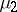and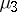are the second and third central moments of the distribution, respectively. For distributions that are symmetric with respect to the mathematical expectation,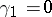; depending on the sign ofone speaks of positive asymmetry () and negative asymmetry (). In the case of the binomial distribution corresponding toBernoulli trials with probability of success,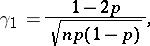(*)

one has: If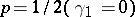, the distribution is symmetric; if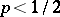or, one obtains typical distribution diagrams with a positive (Fig.a) and negative (Fig.b) asymmetry.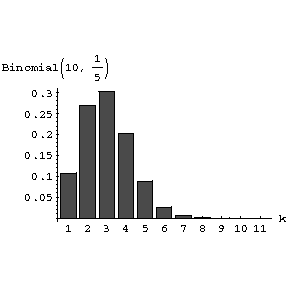Figure: a013590a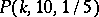. Diagram of the binomial distribution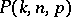corresponding toBernoulli trials, with positive asymmetry (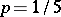).Figure: a013590b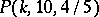. Diagram of the binomial distributioncorresponding to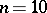Bernoulli trials, with negative asymmetry ().

The asymmetry coefficient (*) tends to zero as, in accordance with the fact that a normalized binomial distribution converges to the standard normal distribution.

The asymmetry coefficient and the excess coefficient are the most extensively used characteristics of the accuracy with which the distribution functionof the normalized sumwhere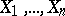are identically distributed and mutually independent with asymmetry coefficient, may be approximated by the normal distribution function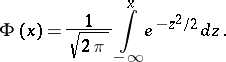Under fairly general conditions the Edgeworth series yields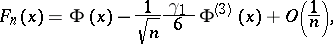whereis the derivative of order three.